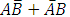# The open-loop transfer function of a unity feedback control system is  The closed loop transfer function will have poles at

1.  -2, -2

2.  -2, -1

3.  -2,±j

4.   -2, 2

4

-2, -2

Explanation :
No Explanation available for this question

# The basic memory cell of dynamic RAM consist of

1.  A capacitance

2.  A transistor

3.  A flip-flop

4.  A transistor acting as a capacitor

4

A transistor acting as a capacitor

Explanation :
No Explanation available for this question

# A periodic rectangular signal, X(t) has the wave from shown in figure below. Frequency of the fifth harmonic of its spectrum is

1.  40 Hz

2.  200 Hz

3.   250 Hz

4.   1250 Hz

4

1250 Hz

Explanation :
No Explanation available for this question

# Which of the following use least power

1.  TTL

2.  ECL

3.  CMOS

4.  All use same power

4

CMOS

Explanation :
No Explanation available for this question

# PROMs are used to save

1.  Bulk information

2.  Sequential information

3.  Information to be accessed rarely

4.  Relatively permanent information

4

Relatively permanent information

Explanation :
No Explanation available for this question

# In the circuit shown in figure below, X is an element which always absorbs power. During a particular operation, it sets up a current of 1 amp in the direction shown and absorbs a power Px. it is possible that X can absorb the same power Px for another current I, the value of this currents is

1.  (3-√14)amps

2.   (3+√14)amps

3.  5 amps

4.   None of these

4

5 amps

Explanation :
No Explanation available for this question

# The state representation of a second order system is 1= --x1+µ x2 = x1-2x2+µ Consider the following statements regarding the above system: 1. The system is completely state controllable 2. If x1 is the output, then the system is completely output controllable 3. If x2 is the output, then the system is completely output controllable. Of these statements:

1.   1, 2 and 3 correct

2.  1 and 2 are correct

3.  2 and 3 are correct

4.   1 and 3 are correct

4

2 and 3 are correct

Explanation :
No Explanation available for this question

# A Water at home is switched on to the a. c. mains supplying power at (230 V/50) Hz. The frequency of instantaneous power consumed by the boiler is

1.  0 Hz

2.  50 Hz

3.  100 Hz

4.  150 Hz

4

100 Hz

Explanation :
No Explanation available for this question

# In the series RC circuit shown in figure below, the voltage across C starts increasing when the d. c. source is switched on. The rate of increase of voltage across C at the instant just after the switch is closed (i. e. at t= 0+), will be

1.  Zero

2.   Infinity

3.  RC

4.  1/ RC

4

1/ RC

Explanation :
No Explanation available for this question

# For the logic circuit shown in the figure, the output Y is given by For the logic circuit shown in the figure, the output Y is given by

1.  A . B

2.

3.

4.

4